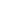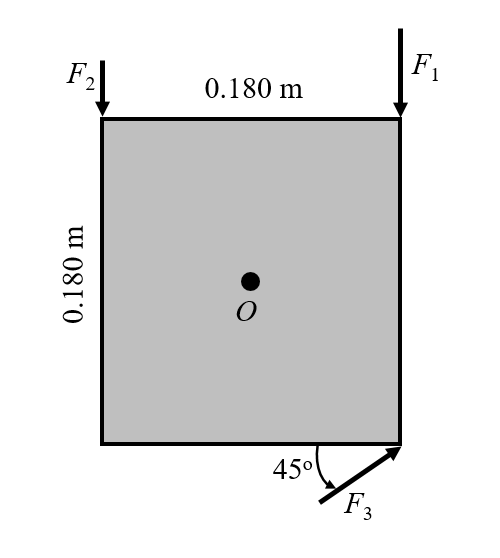Flat 50% off

Ends in

• JEE Main
•• 11th
•• Physics
• Back to Doubt Clearing

## A square metal plate 0.180 m on each side is pivoted about an axis through point $O$ at its centre and perpendicular to the plate (Fig. below). Calculate the net torque about this axis due to the three forces shown in the figure if the magnitudes of the forces are $F_1 = 24.0 N, F_2 = 15.8 N,$ and $F_3 = 15.5 N$. The plate and all forces are in the plane of the page.### Asked By Alamanchili Yudhi Vishnu Bhargav

Updated Thu, 16 Aug 2018 05:35 pm